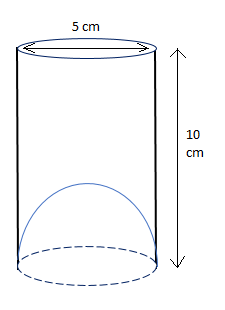QUESTION

# A juice seller was serving his customers using glass. The inner diameter of the cylindrical glass was 5 cm, but the bottom of the glass had a hemispherical raised portion which reduced the capacity of the glass. If the height of the glass was 10 cm, find what the apparent capacity of the glass was and what actual capacity was. $\left( {{\text{Use }}\pi = {\text{3}}{\text{.14}}} \right)$Hint: First of all, find the volume of the total glass which is of the cylindrical form and then find the bottom potion raised in the glass which is of the hemisphere form. Then the apparent volume of the glass is given by the volume of the cylinder and the actual capacity of the glass is the difference of the volumes of cylinder and hemisphere.

Here apparent capacity of the glass = Volume of the cylinder
Actual capacity of the glass = Volume of cylinder – volume of hemisphere
Now consider volume of the cylinder
Given inner diameter of the glass = 5 cm
So, radius $r = \dfrac{{{\text{Diameter}}}}{2} = \dfrac{5}{2} = 2.5{\text{ cm}}$
Height of the glass $h$ = 10 cm
We know that volume of the cylindrical glass with height $h$ and radius $r$is given by ${V_c} = \pi {r^2}h$
So, the volume of the cylinder is given by
$\Rightarrow {V_c} = \pi {\left( {2.5} \right)^2}\left( {10} \right) \\ \Rightarrow {V_c} = 3.14\left( {2.5 \times 2.5} \right)\left( {10} \right) \\ \therefore {V_c} = 196.25{\text{ c}}{{\text{m}}^3} \\$
Now consider the volume of the hemisphere
Radius of hemisphere $r = 2.5{\text{ cm}}$
We know that the Volume of the hemisphere of radius $r$ is given by ${V_h} = \dfrac{2}{3}\pi {r^3}$.
$\Rightarrow {V_h} = \dfrac{2}{3}\pi {r^3} \\ \Rightarrow {V_h} = \dfrac{2}{3}\left( {3.14} \right){\left( {2.5} \right)^3} \\ \Rightarrow {V_h} = \dfrac{2}{3} \times 3.14 \times 2.5 \times 2.5 \times 2.5 \\ \therefore {V_h} = 32.7{\text{ c}}{{\text{m}}^3} \\$
Now,
Apparent capacity of the glass = Volume of the cylinder = $196.25{\text{ c}}{{\text{m}}^3}$
Actual capacity of the glass = Volume of cylinder – volume of hemisphere
$= 196.25 - 32.7 \\ = 163.54{\text{ c}}{{\text{m}}^3} \\$
Hence the apparent capacity of glass is $196.25{\text{ c}}{{\text{m}}^3}$ and actual capacity of the glass is $163.54{\text{ c}}{{\text{m}}^3}$.

Note: The Volume of the cylindrical glass with height $h$ and radius $r$ is given by ${V_c} = \pi {r^2}h$ and the Volume of the hemisphere of radius $r$ is given by ${V_h} = \dfrac{2}{3}\pi {r^3}$. The volume of the bottom portion i.e., volume of the hemisphere is the extra profit made by the juice seller.